# Open Benefit Code

1. First, open2. Select the Benefit Code to update.

3. Press Modify, which will open the Modify – Benefit Codes window.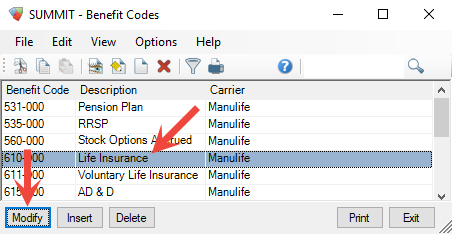Figure 1. Select the Benefit Code, then press Modify.
4. Open the Benefit Data tab from the top of the window.Figure 2. Open the Beenfit Data tab.

# Update Rate Source

## Rate Sources

There are three options for the benefit rate source—Rate Formula, Rate Table, and Fixed Rate. (Only one rate source can be used per benefit code.)Figure 3. Options for setting the benefit rate.
Fixed Rate

Assigns a constant value to the rate (depending on the Premium Formula used, this can be a dollar amount, decimal, or percentage).

Rate Formula

Allows the benefit rate to be calculated from a customized formula. This formula might make use of

• Built-in functions like @MIN, @MAX, @IF

• Mathematical functions like multiplication, division, subtraction, or addition,

• Variables that can draw on employee data automatically.

Rate Table

Can only be used for Life Insurance benefits and changes the rate for the benefit recipient according to the age, gender, and nicotine use data on the Employee Profile.

### Changing Fixed Rate

1. Select Fixed Rate from the Rate (K1) options which will make the number field to the right available.

2. Enter the new fixed rate for the benefit in the number field now.Figure 4. When Fixed Rate is enabled, the rate can be entered as a decimal to the right.
3. Press OK button at the bottom of the window to save the change to the benefit rate.

### Changing Rate Formula

1. First select Rate Formula from the Rate (K1) options.

2. Then select the Rate Formula tab from the top of the Benefit Codes window.Figure 5. When Rate Formula is enabled, the rate must be entered using a formula on the Formula tab.
3. Enter the new benefit rate formula into the formula fieldss (F1F9; usually, formulas will only require using the first field).

 Notes:Formulas entered into the fields (F1, for example) are evaluated, and the result is stored into a variable matching the field (F1).Formula fields are evaluated from top to bottom, and the value of any previous field can be used in equations.
1. Enter the variable used for the Rate (K1) field of the Rate Formula.

2. Press OK at the bottom of the window to save the changes to the benefit rate.

Example 1. Fomula Benefit Rate Example

You might want the rate for each Pay Group to be different. This can be done by using a formula that selects a rate based on the Pay Group directly:

1. The calculation is entered in the F1 field, then the result is stored into a variable (the “F1” variable).

2. The Rate is set to equal F1; meaning the value of the rate will come from the equation entered in the “F1” field.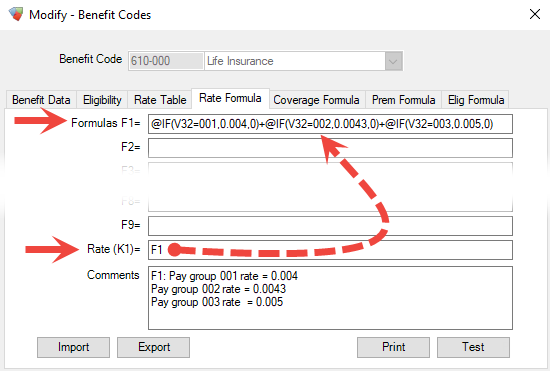Notes:@IF is a built-in function for choosing one of two values using a test (in this case the test checks the Pay Group Code).V32 is a variable for the employee Pay Group.
 More information on the available variables, functions, and examples of some common benefit formulas, are available in the BA: BENEFITS ADMINISTRATION REFERENCE MANUAL under Appendix A and Appendix B.

Existing formulas can be imported and exported using the Import and Export buttons at the bottom of the Modify – Benefit Codes window

### Changing Rate Table

1. Select Rate Table from the Rate (K1) options.

2. Select the Rate Table tab from the top of the Benefit Codes window.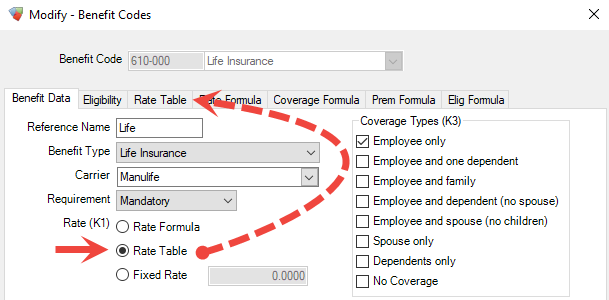Figure 6. When Rate Table is enabled, the rate is entered from the Rate Table tab.
3. Enter the age range, and smoking and non-smoking rate for each row in the Life Insurance benefit rate table.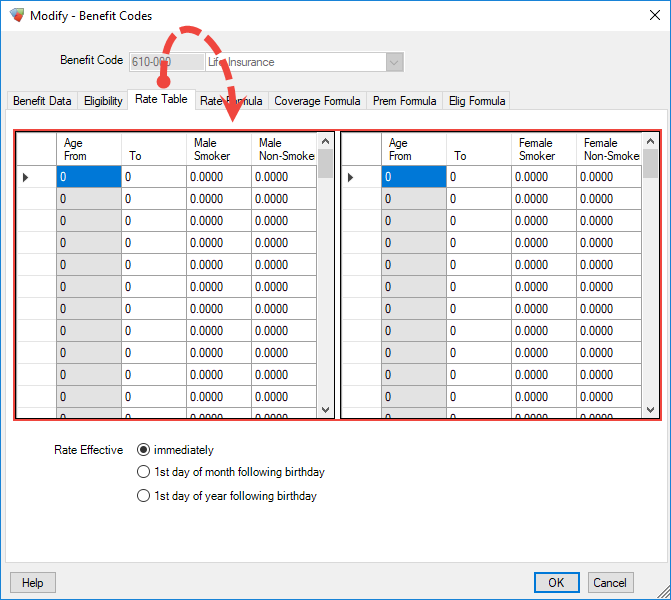Enter the “To” field of an age range and the “Age From” field for the next row will automatically be generated. (You can enter a 0 on the “Age From” field of a row to remove the automatically generated “To” value).The age, sex, and smoking status of the employee is pulled from the Employee Profile automatically and is used to select the appropriate rate value from the table.

During year-end you might need to update the benefit rates for Life Insurance according to a table of rates from your benefit provider:

Table 1. Example Life Insurance Benefit Rates
MONTHLY PREMIUM PER \$25,000 UNIT

Age

Non-Smoker

Standard

MALE

FEMALE

MALE

FEMALE

Under 30

\$ 2.20

\$ 1.70

\$ 3.50

\$ 2.50

30 – 34

2.40

2.00

4.00

3.00

35 – 39

2.90

2.20

5.25

4.00

40 – 44

4.10

3.20

8.00

6.00

45 – 49

6.00

4.60

12.25

9.00

50 – 54

8.90

6.50

18.00

12.25

55 – 59

14.40

9.60

26.75

16.50

60 – 72

16.80

11.80

35.50

20.25

Enter the information from the benefit provider’s table into each row of the Rate Table.

 Tip:You can press Enter to move down a row, or Tab to move to the next column).
1. Enter the upper-end of the age range into the “To” field of each row.
(E.g. for “Under 30” you would enter 29).

2. Enter the provided rates into the appropriate columns.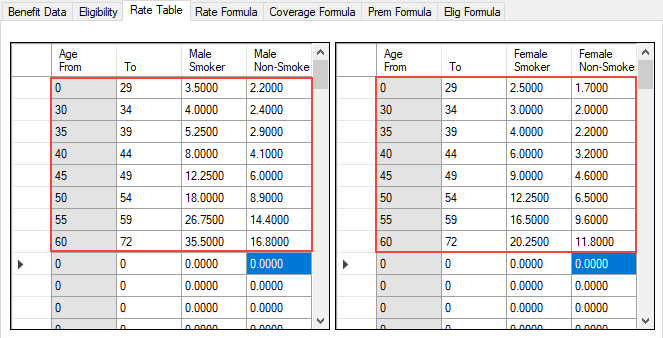Figure 7. Example of the values from Table 1 entered into the Benefit Code `Rate Table`

Did you find it helpful? Yes No

Send feedback